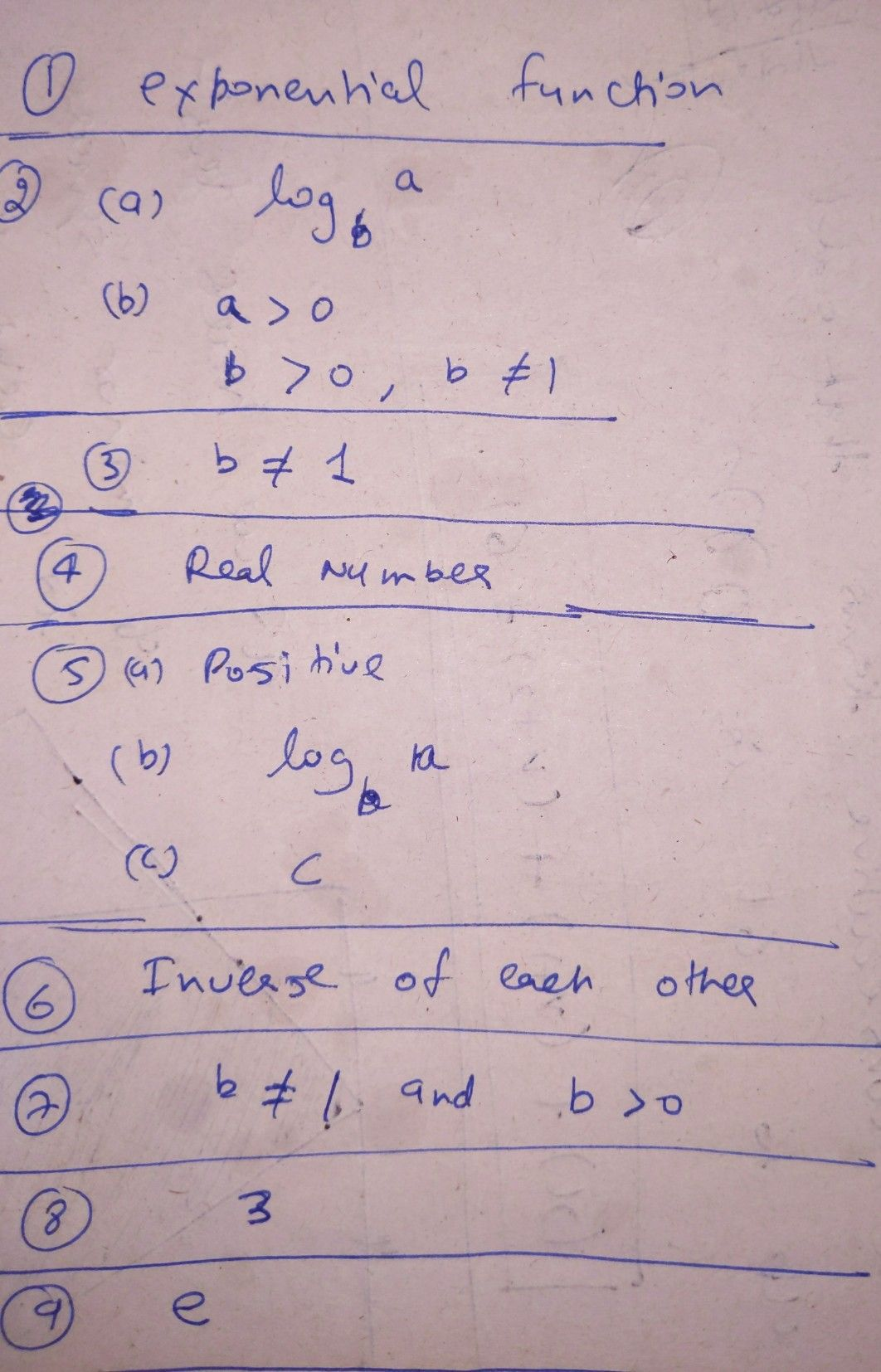Symbol
ProblemLat I Have Serence Learned rmulas. omplete the following statements by writing the $corect$ $Nord$ or $Nox△s$ and 1. Logarithm is the inverse of. 2. The logarithm of a with base b is denoted by. and is defined as $c=log _{b}a$ if and only if in logarithmic form log, $gba$ ,the value of b cannot be 4 The value of log $ba$ can be 5. Let a, b, and $dC$ be real numbers such that $b≠1.Th0$ logarithm of a with base bis denoted by and is defined as if and only $ifa=b^{c}$ 6. Logarithmic functions and exponential functions are 7. In logarithmic form logb $g_{ba,}$ b cannot be 8. The base in the given logarithmic expression $log _{3}5is$ aIf the base is not written in the logarithmic expression, then it is understood to be e given $log _{7}343,$ $1tis$ is the $same$ as asking "What will be the exponent of to $get$ $since$ $7-=343T$ 343Therefore, $log _{7}343$ $=$ then, $log _{7}343=-$ -
10th-13th grade
Other
Search count: 120
SolutionQanda teacher - Poojafeel free to ask if you have any doubt